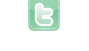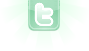This site requires JavaScript, please enable it in your browser!## Loading existing world instances?

I am producing a game for a school assignment, and have decided to make several worlds that the player can traverse by moving to the edge of the screen (like original Zelda pretty much) and want to be able to do this without creating a new instance of a world every time the player enters it. I want to declare an instance of every area once, and load that instance every time that area is accessed, but where and how do i do that? Does anyone have a suggestion for a better way to achieve the same result? Here is what the current code for a world looks like:
```import greenfoot.*;  // (World, Actor, GreenfootImage, Greenfoot and MouseInfo)

/**
* Write a description of class World2 here.
*
* @author (your name)
* @version (a version number or a date)
*/
public class World2 extends World
{

/**
* Constructor for objects of class World2.
*
*/
public World2(int x, int y)
{
// Create a new world with 600x400 cells with a cell size of 1x1 pixels.
super(640, 640, 1);
prepare(x, y);
}

private void prepare(int x, int y)
{
setPaintOrder(Bg2.class, Bg2Mid.class, Player.class);
Bg2 Bg2 = new Bg2();
addObject(Bg2,320,320);

Bg2Mid Bg2Mid = new Bg2Mid();
addObject(Bg2Mid,320,320);

Player Player = new Player();
addObject(Player,x,y);
}

static public void moveWorlds(int edge, int x, int y){
if (edge == 0){
return;
}
if (edge == 1){
Greenfoot.setWorld(new Lake (x, 640));
}
if (edge == 2){
Greenfoot.setWorld(new MyWorld(0, y));
}
if (edge == 3){
Greenfoot.setWorld(new Lake(x, 0));
}
if (edge == 4){
Greenfoot.setWorld(new Lake(640, y));
}
}
}
```
As you can see, I call the moveWorlds() function from my Player class and every world has a constructor that places the player in the appropriate position at the edge, creating a recursive system that begins with the initiation of the first world from the main menu world.
You can create all worlds at the beginning and store them into variables and then, instead of using “new …”, you use the variable. You could even store the worlds in a 2D-array and then pick the world in the correct world when moving to a different one.
Super_Hippo wrote...
You can create all worlds at the beginning and store them into variables and then, instead of using “new …”, you use the variable. You could even store the worlds in a 2D-array and then pick the world in the correct world when moving to a different one.
Where would I put that?
It should be a static variable which is set at the beginning. Where doesn’t really matter as long as you can access it from anywhere needed.
Super_Hippo wrote...
It should be a static variable which is set at the beginning. Where doesn’t really matter as long as you can access it from anywhere needed.
Okay, cool, but I'm still unsure about how i'm going to place the player in the right spot. Currently it's in the constructor of the world.
Probably not very elegant, but I just threw this together and at least it seems to work:
```import greenfoot.*;

public class StartWorld extends World
{
private static Player player;
private static Level[][] worlds = new Level;

public StartWorld()
{
super(600, 400, 1);
player = new Player();

for (int i=0; i<worlds.length; i++) for (int j=0; j<worlds[i].length; j++)
{
worlds[i][j] = new Level(i, j);
}

worlds.addObject(player, 100, 100);
Greenfoot.setWorld(worlds);
}

public static Level getWorld(int x, int y) {return worlds[x][y];}
public static Player getPlayer() {return player;}
}```
```import greenfoot.*;

public class Level extends World
{
private int x, y;

public Level(int x, int y)
{
super(600, 400, 1, false);
this.x=x; this.y=y;

if (x==0)
{
for (int j=20; j<getHeight(); j+=40)
{
addObject(new Tree(), 20, j);
}
}

if (y==0)
{
for (int i=20; i<getWidth(); i+=40)
{
addObject(new Tree(), i, 20);
}
}
}

public void changeLevel(int dx, int dy)
{
World nextLevel = StartWorld.getWorld(x+dx, y+dy);
Actor player = StartWorld.getPlayer();
nextLevel.addObject(player, dx==0 ? player.getX() : nextLevel.getWidth()-player.getX()-1, dy==0 ? player.getY() : nextLevel.getHeight()-player.getY()-1);
Greenfoot.setWorld(nextLevel);
}
}```
```import greenfoot.*;

public class Player extends Actor
{
private int speed = 2;

public Player()
{

}

public void act()
{
int dx=0, dy=0;
if (Greenfoot.isKeyDown("up")) dy--;
if (Greenfoot.isKeyDown("down")) dy++;
if (Greenfoot.isKeyDown("left")) dx--;
if (Greenfoot.isKeyDown("right")) dx++;
if (dx != 0 || dy != 0)
{
setLocation(getX()+dx*speed, getY()+dy*speed);
if (isTouching(Tree.class))
{
setLocation(getX()-dx*speed, getY()-dy*speed);
}
}

if (getX()<0) ((Level) getWorld()).changeLevel(-1, 0);
else if (getX()>getWorld().getWidth()-1) ((Level) getWorld()).changeLevel(1, 0);
else if (getY()<0) ((Level) getWorld()).changeLevel(0, -1);
else if (getY()>getWorld().getHeight()-1) ((Level) getWorld()).changeLevel(0, 1);
}
}```
```import greenfoot.*;

public class Tree extends Actor
{

}```
this is awesome!! thank you so much!
You need to login to post a reply.